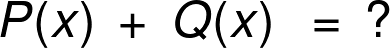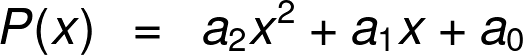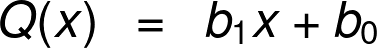# Adding and Subtracting Polynomials Calculator

Created by Maciej Kowalski, PhD candidate
Reviewed by Steven Wooding
Last updated: May 18, 2023

Welcome to Omni's adding and subtracting polynomials calculator, where we'll learn to find the sum and difference of polynomials. In fact, the operation is fairly simple, much simpler than polynomial multiplication. Nevertheless, let's go through it slowly, step by step, to get a clear understanding of the process. And once we know how to add polynomials and how to subtract polynomials, we'll go through a nice example at the end.

If you decide to multiply polynomials, do try our multiplying polynomials calculator.

## Polynomials, monomials, binomials

Ever since we've gone past the point of counting apples and candies, we've been handling polynomials without even knowing it. The moment we first met geometry and laid our eyes on the formula for the area of a square, our world changed and would never go back to what it had been. After all, mathematics decided to get letters involved.

In algebra, those letters are known as variables. They represent an object (usually a number) that we don't know or don't want to specify at that moment. They are especially useful in formulas that describe laws that govern our world and the dependence between different values. For instance, the equation A = πr² uses variables A, r, and π, where only π is determined beforehand. The other two represent not a specific number but a universal quantity: the area of a circle and the length of its radius, respectively.

A polynomial is an algebraic expression (not an equation, mind you!) in which the variables appear only in non-negative integer powers. In other words, the quantity cannot contain things like radicals, logarithms, or trigonometric functions with letters inside them. Below we list a few examples of polynomials:

• x + 2y

• a² + 2ab + b

• πr²

• n³ - 0.7n + ⅜

• 1 + 3 + x⁵ - x⁷ + 19x⁵

Binomials and monomials are special cases of polynomials: they are those with two and one terms, respectively (as suggested by the prefixes bi- and mono- meaning double and single). As such, the first of the above is an example of a binomial, while the third is a monomial. There are also trinomials, which have (surprise, surprise!) three terms, but they're not nearly as common as the other two and appear mostly in quadratic equations. In case you want to learn more about trinomials, we'd suggest checking out our factoring trinomials calculator.

Now that we know what objects we're dealing with, it's time to get down to business and introduce some operations. First of all, we'll see how to add polynomials.

Just like in arithmetics, finding the sum in the new setting is a fairly easy task. If we were to, say, divide polynomials, the matter would get significantly harder. However, we can enjoy the simplicity of addition and even make the task extra simple with our adding polynomials calculator.

The basic rule on how to add polynomials is: combine only similar terms. To make it more mathematically precise, we can only add monomials with the same variables and variable powers (but possibly, different constants before them). For instance, we can add 3xy² to -0.5xy², but not to 2x²y² since the power of x is different (2 instead of 1). Not to mention terms that have different letters: you can never group those into one.

Let us mention here that our adding and subtracting polynomials calculator deals only with expressions that have one variable (in our tool, it's x) and at most in power 6. Such objects are most common in algebra, and they're enough to explain the concept.

Suppose that we have two such polynomials P(x) = a₅x⁵ + a₄x⁴ + a₃x³ + a₂x² + a₁x + a₀ and Q(x) = b₄x⁴ + b₃x³ + b₂x² + b₁x + b₀ and you want to add them. Note how their degrees (the maximal powers of x) are 5 and 4, respectively. So, what is the sum of the polynomials?

As mentioned above, we can add only terms that have the same powers of the variable, which means that, for instance, we combine a₃x³ with b₃x³ and nothing else. And the combining means simply adding the numbers in front:

P(x) + Q(x) = (a₅x⁵ + a₄x⁴ + a₃x³ + a₂x² + a₁x + a₀) + (b₄x⁴ + b₃x³ + b₂x² + b₁x + b₀)

= a₅x⁵ + (a₄ + b₄)x⁴ + (a₃ + b₃)x³ + (a₂ + b₂)x² + (a₁ + b₁)x + (a₀ + b₀).

Observe how the first summand has only a₅ in front since there was no term with x⁵ in Q(x).

And that's all there is. Easy, wasn't it? And we can always count on Omni's adding polynomials calculator to do it all for us.

Our polynomial graphing calculator helps us understand how to graph polynomial functions. Letting you know if you would ever need to do so.

## How to subtract polynomials?

Subtracting polynomials is to adding polynomials what subtracting numbers is to adding numbers. Well, that sentence didn't clarify much, did it? Let's try to phrase it differently.

Subtracting polynomials is as easy as adding them; we simply change sums to differences throughout. To illustrate this, let's see how to subtract polynomials P(x) = a₅x⁵ + a₄x⁴ + a₃x³ + a₂x² + a₁x + a₀ and Q(x) = b₄x⁴ + b₃x³ + b₂x² + b₁x + b₀. The rule is the same as in the above section: we're only allowed to combine alike terms. And combining means finding the difference of the coefficients:

P(x) - Q(x) = (a₅x⁵ + a₄x⁴ + a₃x³ + a₂x² + a₁x + a₀) - (b₄x⁴ + b₃x³ + b₂x² + b₁x + b₀)

= a₅x⁵ + (a₄ - b₄)x⁴ + (a₃ - b₃)x³ + (a₂ - b₂)x² + (a₁ - b₁)x + (a₀ - b₀).

The formula looks the same, but we need to be careful with the signs. Addition is commutative, so it didn't matter much in which order we put the summands (after all, there were only pluses there). On the other hand, subtraction is not, so minuses must appear only in front of the b terms, i.e., the coefficients of the subtrahend. Remember that if you want to play it extra safe, you can always turn to Omni's subtracting polynomials calculator for help.

That concludes our swift adventure of algebraic theory. We now change the symbols into numbers (although not all, x is here to stay) and lay our hands on a nice example.

Our tools not only help you add, subtract and multiply polynomials but also divide them. But for that one, you would have to refer to our polynomial division calculator.

## Example: using the adding and subtracting polynomials calculator

Let's try out both options in the adding and subtracting polynomials calculator, i.e., we'll find both the sum and difference of P(x) and Q(x) with:

• P(x) = 4x⁴ - x³ + 5x + 1

• Q(x) = x⁵ + 4x⁴ - 7x³ - 3x² + x + 12

First of all, we put our tool to the test. Note how at the top of it, we can choose what we want to find: the sum or the difference by choosing respectively "add" or "subtract" in "I want to ... polynomials."

Next, we need to specify the degrees of P(x) and Q(x). We see that the maximal exponents of x in the two are 4 and 5, respectively, so we choose the numbers from the list in the corresponding fields. That will trigger formulas for the two polynomials with the notation used underneath:

• P(x) = a₄x⁴ + a₃x³ + a₂x² + a₁x + a₀

• Q(x) = b₅x⁵ + b₄x⁴ + b₃x³ + b₂x² + b₁x + b₀

Looking back at the two expressions we have, we see that we need to input:

a₄ = 4, a₃ = -1, a₂ = 0, a₁ = 5, a₀ = 1; and

b₅ = 1, b₄ = 4, b₃ = -7, b₂ = -3, b₁ = 1, b₀ = 12.

Take a minute to pay special care of the following things:

• Symbols a₃ and b₃ are negative because we have minuses in the corresponding places in the polynomials.

• We have a₀ = 1, b₅ = 1, and b₁ = 1 even though there are no numbers in the corresponding places in P(x) and Q(x). That is because, by convention, if the coefficient is 1, we don't write it. For a similar reason, we have a₃ = -1 with the minus.

• We input a₂ = 0 because P(x) has no term with , which, in fact, means that its coefficient is equal to 0.

The moment we input the last variable, the adding and subtracting polynomials calculator will spit out the answer underneath. However, before we reveal it, let's see how to add and subtract the polynomials ourselves.

We apply the formulas from the above sections. So what is the sum of the polynomials?

P(x) + Q(x) = (4x⁴ - x³ + 5x + 1) + (x⁵ + 4x⁴ - 7x³ - 3x² + x + 12)

= x⁵ + (4 + 4)x⁴ + ((-1) + (-7))x³ - 3x² + (5 + 1)x + (1 + 12)

= x⁵ + 8x⁴ - 8x³ - 3x² + 6x + 13.

We finish by showing how to subtract the two polynomials:

P(x) - Q(x) = (4x⁴ - x³ + 5x + 1) + (x⁵ + 4x⁴ - 7x³ - 3x² + x + 12)

= -x⁵ + (4 - 4)x⁴ + ((-1) - (-7))x³ + 3x² + (5 - 1)x + (1 - 12)

= -x⁵ + 6x³ + 3x² + 4x - 11.

Voilà! It wasn't so bad, was it?

Maciej Kowalski, PhD candidateI want to
polynomials
Polynomial P(x)
Degree
2a₂
a₁
a₀
Polynomial Q(x)
Degree
1b₁
b₀
People also viewed…

### Black Friday

How to get best deals on Black Friday? The struggle is real, let us help you with this Black Friday calculator!

### Least common factor

Our least common factor calculator will help you find the smallest multiple common to a set of numbers.

### Snowman

The perfect snowman calculator uses math & science rules to help you design the snowman of your dreams!

### Theta

With Omni's theta calculator, you will learn how to solve every trigonometric function for any angle theta!